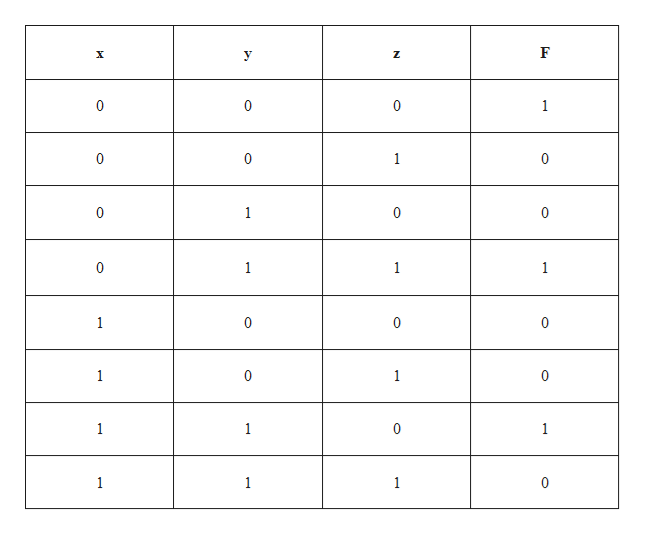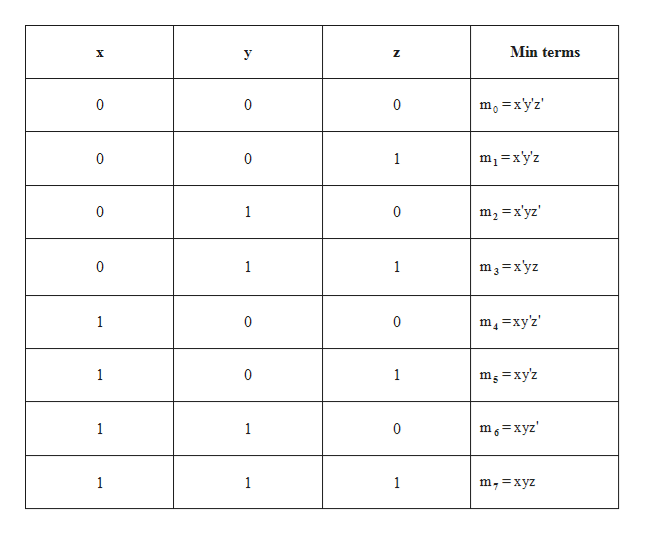# Using the following truth table, write the equivalent Boolean equations in Sum of Product and Product of Sum formsxyzF00010010010001111000101011011110

Question
1. Using the following truth table, write the equivalent Boolean equations in Sum of Product and Product of Sum forms
 x y z F 0 0 0 1 0 0 1 0 0 1 0 0 0 1 1 1 1 0 0 0 1 0 1 0 1 1 0 1 1 1 1 0

check_circleExpert Solution
Step 1

Simplification of Sum Of Products (SOP):

The truth table of the SOP is as followshelp_outlineImage Transcriptionclose0 0 0 1 0 0 1 0 1 0 0 1 1 0 1 1 0 0 0 1 1 0 0 1 1 C 1 1 1 1 0 N fullscreen
Step 2

The min terms for the given values is as follows.help_outlineImage TranscriptioncloseMin terms X mo =xy'z 0 0 0 m-xy'z 0 0 1 1 m2 x'yz' 0 C m3=x'yz 0 1 1 m4=xy'2 1 C 0 1 0 1 mg=xyz m6=xyz 1 1 C 1 1 1 m,=xyz fullscreen
Step 3

The SOP form can be written as F=∑(m0+m4+m6)

By expanding the above equation, we get, F= m0+m4+m6

By substituting the respective min terms in the above equation, ...

### Want to see the full answer?

See Solution

#### Want to see this answer and more?

Solutions are written by subject experts who are available 24/7. Questions are typically answered within 1 hour*

See Solution
*Response times may vary by subject and question
Tagged in

### Boolean Logic